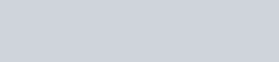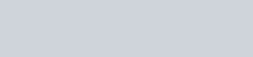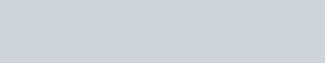Solve the following system of linear equation graphically and shade the region between the two lines and x-axis:
Question:

Solve the following system of linear equation graphically and shade the region between the two lines and x-axis:

(i) $2 x+3 y=12$,

$x-y=1$

(ii) $3 x+2 y-4=0$,

$2 x-3 y-7=0$

(iii) $3 x+2 y-11=0$

$2 x-3 y+10=0$

Solution:

(i) The given equations are:

$2 x+3 y=12$ ….(i)

$x-y=1$….(ii)

Putting $x=0$ in equation $(i)$, we get:

$\Rightarrow 2 \times 0+3 y=12$

$\Rightarrow y=4$

$x=0, \quad y=4$

Putting $y=0$ in equation $(i)$ we get:

$\Rightarrow 2 x+3 \times 0=12$

$\Rightarrow x=6$

$x=6, \quad y=0$

Putting $y=0$ in equation $(i)$ we get:

$\Rightarrow 2 x+3 \times 0=12$

$\Rightarrow x=6$

$x=6, y=0$

Use the following table to draw the graph.Draw the graph by plotting the two points $A(0,4), B(6,0)$ from table$x-y=1$ ……(ii)

Putting $x=0$ in equation $(i i)$ we get:

$\Rightarrow 0-y=1$

$\Rightarrow y=-1$

$x=0, \quad y=-1$

Putting $y=0$ in equation $(i i)$, we get:

$\Rightarrow x-0=1$

$\Rightarrow x=1$

$x=1, \quad y=0$

Use the following table to draw the graph.Draw the graph by plotting the two points $C(0,-1), D(1,0)$ from table.

The two lines intersect at $P(3,2)$. The region enclosed by the lines represented by the given equations and $x$-axis are shown in the above figure

Hence, $x=3$ and $y=2$ is the solution.

(ii) The given equations are:

$3 x+2 y-4=0$….(i)

$2 x-3 y-7=0$….(ii)

Putting $x=0$ in equation $(i)$, we get:

$\Rightarrow 3 \times 0+2 y=4$

$\Rightarrow y=2$

$x=0, y=2$

Putting $y=0$ in equation $(i)$ we get:

$\Rightarrow 3 x+2 \times 0=4$

$\Rightarrow x=4 / 3$

$x=4 / 3, y=0$

Use the following table to draw the graph.The graph of (i) can be obtained by plotting the two points $A(0,2), B(4 / 3,0)$.

$2 x-3 y-7=0$…..(ii)

Putting $x=0$ in equation (ii) we get:

$\Rightarrow 2 x-3 \times 0=7$

$\Rightarrow x=7 / 2$

$x=7 / 2, y=0$

Use the following table to draw the graph.Draw the graph by plotting the two points $C(0,-7 / 3), D(7 / 2,0)$ from table.The two lines intersect at $P(2,-1)$. The area enclosed by the lines represented by the given equations and the coordinates $x$-axis and shaded the area in graph.

Hence, $x=2$ and $y=-1$ is the solution.

(iii) The given equations are:

$3 x+2 y-11=0$$\ldots \ldots(i) 2 x-3 y+10=0$$\ldots(i i)$

Putting $x=0$ in equation $(i)$, we get:

$\Rightarrow 3 \times 0+2 y=11$

$\Rightarrow y=11 / 2$

$x=0, \quad y=11 / 2$

Putting $y=0$ in equation $(i)$ we get:

$\Rightarrow 3 x+2 \times 0=11$

$\Rightarrow x=11 / 3$

$x=11 / 3, y=0$

Use the following table to draw the graph.Draw the graph by plotting the two points $A(0,11 / 2), B(11 / 3,0)$ from table$2 x-3 y+10=0 \quad \ldots \ldots \ldots .(i i)$

Putting $x=0$ in equation $(i i)$ we get:

$\Rightarrow 2 \times 0-3 y=-10$

$\Rightarrow y=10 / 3$

$x=0, y=10 / 3$

Putting $y=0$ in equation $(i i)$, we get:

$\Rightarrow 2 x-3 \times 0=-10$

$\Rightarrow x=-5$

$x=-5, \quad y=0$

Use the following table to draw the graph.Draw the graph by plotting the two points $C(0,10 / 3), D(-5,0)$ from table.

The two lines intersect at $P(1,4)$. The area enclosed by the lines represented by the given equations and the coordinates $x$-axis and shaded the area in graph.

Hence, $x=1$ and $y=4$ is the solution.